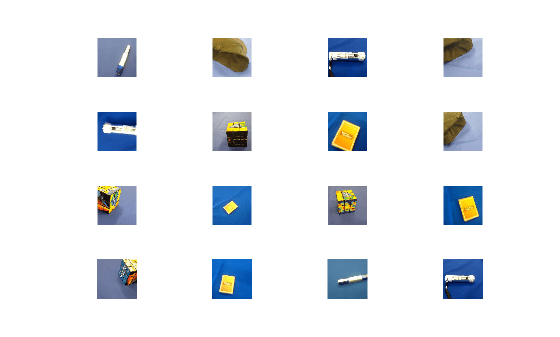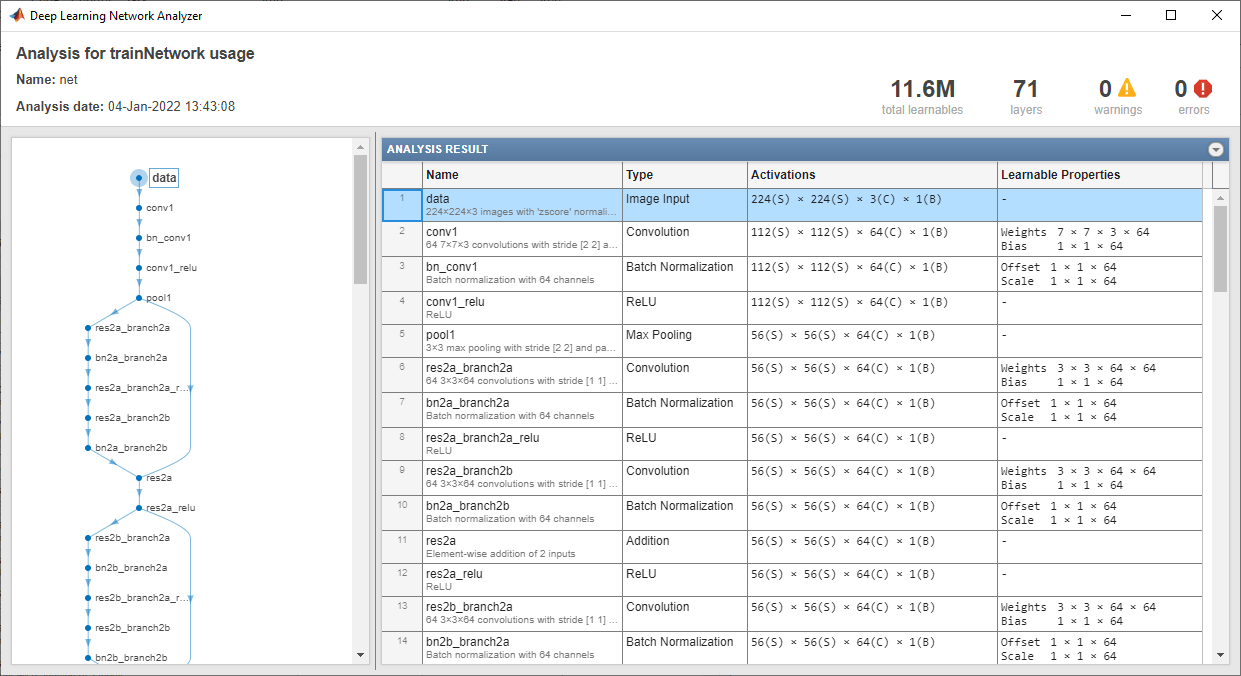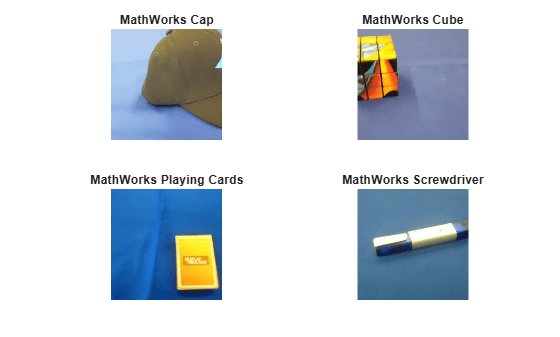# 使用预训练网络提取图像特征

### 加载数据

```unzip('MerchData.zip'); imds = imageDatastore('MerchData','IncludeSubfolders',true,'LabelSource','foldernames'); [imdsTrain,imdsTest] = splitEachLabel(imds,0.7,'randomized');```

```numTrainImages = numel(imdsTrain.Labels); idx = randperm(numTrainImages,16); figure for i = 1:16 subplot(4,4,i) I = readimage(imdsTrain,idx(i)); imshow(I) end```### 加载预训练网络

`net = resnet18`
```net = DAGNetwork with properties: Layers: [71x1 nnet.cnn.layer.Layer] Connections: [78x2 table] InputNames: {'data'} OutputNames: {'ClassificationLayer_predictions'} ```

```inputSize = net.Layers(1).InputSize; analyzeNetwork(net)```### 提取图像特征

```augimdsTrain = augmentedImageDatastore(inputSize(1:2),imdsTrain); augimdsTest = augmentedImageDatastore(inputSize(1:2),imdsTest);```

```layer = 'pool5'; featuresTrain = activations(net,augimdsTrain,layer,'OutputAs','rows'); featuresTest = activations(net,augimdsTest,layer,'OutputAs','rows'); whos featuresTrain```
``` Name Size Bytes Class Attributes featuresTrain 55x512 112640 single ```

```YTrain = imdsTrain.Labels; YTest = imdsTest.Labels;```

### 拟合图像分类器

`classifier = fitcecoc(featuresTrain,YTrain);`

### 对测试图像进行分类

`YPred = predict(classifier,featuresTest);`

```idx = [1 5 10 15]; figure for i = 1:numel(idx) subplot(2,2,i) I = readimage(imdsTest,idx(i)); label = YPred(idx(i)); imshow(I) title(char(label)) end````accuracy = mean(YPred == YTest)`
```accuracy = 1 ```

### 基于较浅特征训练分类器

```layer = 'res3b_relu'; featuresTrain = activations(net,augimdsTrain,layer); featuresTest = activations(net,augimdsTest,layer); whos featuresTrain```
``` Name Size Bytes Class Attributes featuresTrain 28x28x128x55 22077440 single ```

```featuresTrain = squeeze(mean(featuresTrain,[1 2]))'; featuresTest = squeeze(mean(featuresTest,[1 2]))'; whos featuresTrain```
``` Name Size Bytes Class Attributes featuresTrain 55x128 28160 single ```

```classifier = fitcecoc(featuresTrain,YTrain); YPred = predict(classifier,featuresTest); accuracy = mean(YPred == YTest)```
```accuracy = 0.9500 ```

## 另请参阅

(Statistics and Machine Learning Toolbox) |